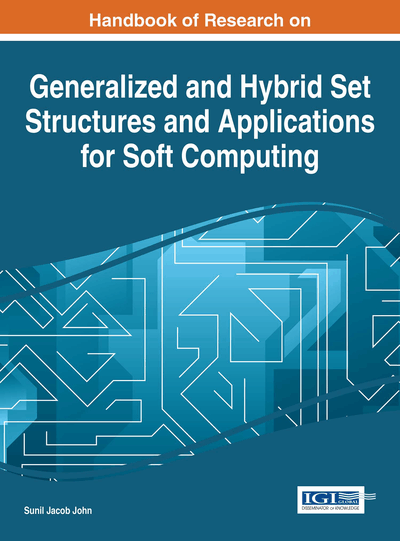# Sequences, Nets, and Filters of Fuzzy Soft Multi Sets in Fuzzy Soft Multi Topological Spaces

Anjan Mukherjee (Tripura University, India) and Ajoy Kanti Das (Iswar Chandra Vidyasagar College, India)
Copyright: © 2016 |Pages: 19
DOI: 10.4018/978-1-4666-9798-0.ch009
OnDemand PDF Download:
Available
\$37.50
No Current Special Offers

## Abstract

In this chapter, the authors introduce a new sequence of fuzzy soft multi sets in fuzzy soft multi topological spaces and their basic properties are studied. The concepts of subsequence, convergence sequence and cluster fuzzy soft multi sets of fuzzy soft multi sets are proposed. Actually Cluster analysis or clustering is the task of grouping a set of objects in such a way that objects in the same group (called a cluster) are more similar to each other than to those in other groups (clusters). It is a main task of exploratory data mining and a common technique for statistical data analysis used in many fields including machine learning, pattern recognition, image analysis, information retrieval and bioinformatics. Here the authors define the notions of net and filter and establish the correspondence between net convergence and filter convergence in fuzzy soft multi topological spaces.
Chapter Preview
Top

## 2. Preliminaries

### 2.1 FUZZY SET THEORY

Zadeh (1965) introduced the concept of fuzzy sets as a new mathematical tool for modeling uncertainty and it made its own place in decision making problems. The word fuzzy means “vagueness”. Fuzzy sets have been introduced by Zadeh (1965) as an extension of the classical characteristic function notation of set. Let A be a crisp set defined over the universe X. Then for any element x in X, either x is a member of A or not. In fuzzy set theory, this property is generalized. Zadeh extended the range of the membership function to the closed interval [0, 1] from two element set {0, 1}.

Definition ( Zadeh,1965): If X is a collection of objects then a fuzzy set A in X is a set of ordered pairs: A={(x, μA(x)) /x ∈ X} where μA(x) is called the membership function of x in A which maps X to the membership space [0,1]. i.e., μA: X →[0,1] .

The words like “young”, “tall”, “good” or “high” are fuzzy concepts. There is no single quantitative value which defines the term “young”, “good” or “high”.

Let A and B be fuzzy sets on a universal set X, with the grade of membership of x in A and B denoted by μA and μB respectively. Zadeh (1965) defined the following relations and operations.

• A = BμA(x) = μB(x) for all x in X;

• ABμA(x) ≤ μB(x) for all x in X;

• μA’ (x) = 1-μA(x) for all x in X; A’ is the complement of A.

• μA∪B (x) = Max{μA(x), μB (x) } for all x in X;

• μA∩B (x) = Min{μA(x), μB (x) } for all x in X;

## Complete Chapter List

Search this Book:
Reset